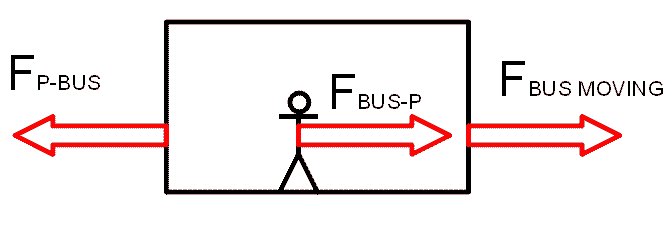# Person in a bus (accelerating system)

pinsky
Hy

I'm having some issues about the Newtons third law.

The image should represent a person in a bus with and the forces acting on them.
The bus and the person are observed from outside the bus. The bus starts accelerating because of the force $F_{BUS MOVING}$. This force represent the resultant force of the friction between the bus and the ground, and the buses starting force.

As a result, friction force $F_{P-BUS}$ occurs and it acts on the bus because of the mass of the person.
As a reaction, a force of the same size but opposite direction acts on the person $F_{BUS-P}$

What is the sum of the forces on the bus?
$F_{BUS MOVING}-F_{P-BUS}$ ?

How would one calculate $F_{P-BUS}$ by knowing the mass of the person and $\mu$?

Is this the solution?
$F_{BUS MOVING}-F_{P-BUS}=F_{BUS-P}$

Since in this case, the bus and the person have the same value and orientation. That is what a observer from the street would see, right?#### Attachments

Last edited:

Homework Helper
Welcome to PF!

Hi pinsky! Welcome to PF!(try using the X2 tag just above the Reply box)

FP-BUS always equals (minus) FBUS-P.

If you regard the friction as an internal force, then you ignore it, and F = (MBUS + m)a.

If you regard the friction as an external force, then you include it, and F - FP-BUS = MBUSa.pinsky
Thanks for the welcome.

I didn't understand your explanation though.

Could you perhaps draw all the forces in a situation where the bus is starting, and the friction between the bus and the person in the bus isn't enough to prevent the person from gently sliding. So in that case we cant observe the bus and the person as a single object.
Draw the forces as they appear from the point of view of a person standing still outside the bus.

I made you a template to spare the time :)

#### Attachments

G = mPaP